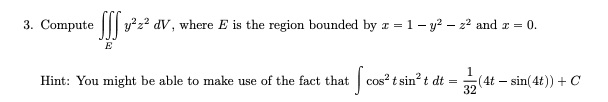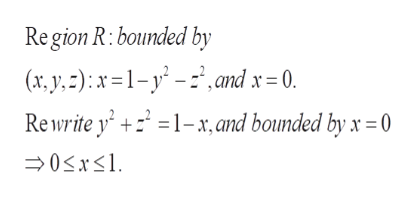# 3. Compute y dV, where E is the region bounded by z = 1 -y2 - z2 and r (E1Cos2 tsin2 t dt(4t - sin(4t))C32Hint: You might be able to make use of the fact that

Question

3. Compute E y 2 z 2 dV , where E is the region bounded by x  1  y 2  z 2 and x help_outlineImage Transcriptionclose3. Compute y dV, where E is the region bounded by z = 1 -y2 - z2 and r ( E 1 Cos2 tsin2 t dt (4t - sin(4t))C 32 Hint: You might be able to make use of the fact that fullscreen
check_circle

Step 1

Region of integration in terms of x,y,z coordinateshelp_outlineImage TranscriptioncloseRe gion R: bounded by (r,y,):x1-y- and x = 0. Re write y' 1-x, and bounded by x = 0 fullscreen
Step 2

The region E in pola...

### Want to see the full answer?

See Solution

#### Want to see this answer and more?

Solutions are written by subject experts who are available 24/7. Questions are typically answered within 1 hour.*

See Solution
*Response times may vary by subject and question.
Tagged in

### Math